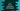# C++ fill_n() function explanation with example## C++ fill_n() function explanation with example:

fill_n is an important method in C++. This method is used to fill upto n positions from a starting position in a container. It accepts one integer or the starting position, the number of positions to fill i.e. n and the value to fill in.

In this post, we will learn how to use fill_n, its syntax and example of fill_n.

### How to use fill_n() function:

To use fill_n, you need to import or include the header. You can also use <bits/stdc++.h> header.

### syntax of fill_n:

fill_n is defined as like below:

``std::fill_n(iterator s, n, v)``

#### Parameters:

It takes three parameter: s, n and v.

• s is an iterator that points to the start position, i.e. it defines the position from where we need to start the filling.
• n is the number of positions to fill.
• v is the value we are filling.

#### Return value of fill_n:

fill_n method returns void, i.e. it doesn’t return anything.

### Example of fill_n:

Let’s try to use fill_n with an example. We will use vector in this example.

``````#include <iostream>
#include <algorithm>
#include <vector>

using namespace std;

int main()
{
vector<char> givenVector(5);

fill_n(givenVector.begin(), 5, '*');

for(char c: givenVector)
cout<<c<<" ";

return 0;
}``````

Here,

• givenVector is a vector that can hold characters and its size is 5.
• We are using fill_n on this vector.
• It starts from the beginning of the vector, i.e. the iterator is starting from the start of the vector
• It fills 5 values of the vector
• The fill character is *.
• The for loop iterates through the characters of the vector one by one and prints them.

#### Output:

If you run this program, it will print the below output:

``* * * * *``

As you can see, all positions are filled with * in the vector.

## Example of fill_n from a specific index:

We can also fill the values of the vector from a specific position. Let’s take a look at the below example:

``````#include <iostream>
#include <algorithm>
#include <vector>
using namespace std;

int main()
{
vector<int> v(8);

fill_n(v.begin() + 3, 5, -3);

for(int i = 0; i< v.size(); i++)
cout<<v[i]<<' ';

return 0;
}``````

Here,

• We are using fill_n to fill integers from index 3 and it is filling 5 positions for the vector v.
• v is a vector that can hold only integer values.
• The for loop is printing the values of the vector.

If you run this program, it will print the below output:

``0 0 0 -3 -3 -3 -3 -3``